Courses

# Test: Thermodynamic Principles Of Metallurgy Ellingham's Diagram & Its Application

## 25 Questions MCQ Test Chemistry Class 12 | Test: Thermodynamic Principles Of Metallurgy Ellingham's Diagram & Its Application

Description
This mock test of Test: Thermodynamic Principles Of Metallurgy Ellingham's Diagram & Its Application for Class 12 helps you for every Class 12 entrance exam. This contains 25 Multiple Choice Questions for Class 12 Test: Thermodynamic Principles Of Metallurgy Ellingham's Diagram & Its Application (mcq) to study with solutions a complete question bank. The solved questions answers in this Test: Thermodynamic Principles Of Metallurgy Ellingham's Diagram & Its Application quiz give you a good mix of easy questions and tough questions. Class 12 students definitely take this Test: Thermodynamic Principles Of Metallurgy Ellingham's Diagram & Its Application exercise for a better result in the exam. You can find other Test: Thermodynamic Principles Of Metallurgy Ellingham's Diagram & Its Application extra questions, long questions & short questions for Class 12 on EduRev as well by searching above.
QUESTION: 1

### Only One Option Correct Type Direction : This section contains 9 multiple choice questions. Each question has four choices (a), (b), (c) and (d), out of which ONLY ONE is correct. Q.  Ellingham diagram represents a graph of

Solution:

Eilingham diagram represents a graph of ΔG° vs T.

QUESTION: 2

### ΔG° vsT plot in the Ellingham diagram slopes down for the reaction.

Solution:

In the case of carbon reacting with dioxygen.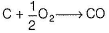two volumes of CO for every one volume of dioxygen used. Thus, ΔS is positive and hence ΔG becomes increasilngly negative as temperature increases. Consequently the line on Eilingham diagram slope downwards.

QUESTION: 3

### The following reactions take place in the blast furnace in the preparation of impure iron. Identify the reaction pertaining to the formation of the slag.

Solution:

A slag is an easily fusible material which is formed when gangue still present in the roasted or the calcined ore combines  with the flux. For example, in the metallurgy of iron, CaO (flux) combines with silica gangue to form easily fusible calcium silicate (CaSiO3) slag.
CaO + SiO2 → CaSiO3 (slag)

QUESTION: 4

Which metal has a greater tendency to form metal oxide?

Solution:

Because the change in free energy i.e. ΔG is highly negative for this reaction,

QUESTION: 5

The main reaction occurring in blast furnace during the extraction of iron from haematite is

Solution:

3Fe2O3 + CO → 2Fe3O4 + CO2
Fe3O4 + CO → 3FeO + CO2
FeO + CO → Fe + CO2

QUESTION: 6

Consider the following reactions at 10000° C,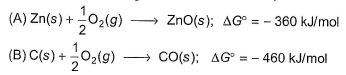Then choose the correct statement from the following.

Solution:

Because the ΔG° of the formation of CO is more negative than that of ZnO at 10000°C.

QUESTION: 7

According to Ellingham diagrams the oxidation reaction of carbon and carbon monoxide may be used to reduce which one of the following oxides at the lowest temperature?

Solution:

Because ΔG° for reductions of rest three oxides [Al2O3, ZnO and MgO] are less negative than that of Cu2O at lower temperature.

QUESTION: 8

The minimum voltage required to electrolyse alumina in the Hall-Heroult process is [Given,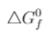(Al2O3) = - 1520 kJ/mol and(CO2) = 394 k J/mol]

Solution:

In Hall - Heroult process the following reactions occur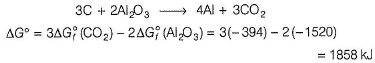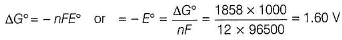QUESTION: 9

Based on the given information(CaO) = - 604.2 kJ/mol and(Al2O3) = - 1582 kJ/mol, which of the following is feasible?

Solution:

3Ca + Al2O→ 2AI + 3CaO
ΔG°= 3 x(CaO) -(Al2O3)
= 3 x (-604.2)-(-1582)
= -1812 + 1582 = -230 kJ
ΔG° is negative. Hence, this reaction is feasible.

*Multiple options can be correct
QUESTION: 10

One or More than One Options Correct Type

Direction : This section contains 5 multiple choice questions. Each question has four choices (a), (b), (c) and (d), out of which ONE or MORE THAN ONE are correct.

Q.

For the metallurgical process of which of the calcined ore can be reduced by carbon?

Solution:

Calcined ore of haematite (Fe2O3) and zincite (ZnO) can be reduced by carbon.

*Multiple options can be correct
QUESTION: 11

For which oxide formation ΔG° is more negative than Gr2O3?

Solution:

According to Eilingham diagram,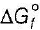for Al2O3 and MgO is more negative than Cr2O3.

*Multiple options can be correct
QUESTION: 12

In the extraction of iron from haematite in blast furnace reducing agent

Solution:
*Multiple options can be correct
QUESTION: 13

Based on the given information,(CaO) = -604.2 kJ/mol and(Al2O3) = - 1582 kJ/mol, which o f the following is correct?

Solution:

3CaO + 2AI → Al2O3 + 3Ca
ΔG °=(Al2O3) - 3(CaO)
= - 1582 - 3 (-604)
= -1582 + 1812 = +230 kJ
ΔG° is positive. Hence this reaction is not spontaneous.

*Multiple options can be correct
QUESTION: 14

Consider the Ellingham diagram given below. Which metal(s) in the diagram can be extracted at 1100 K using coke as a reducing agent?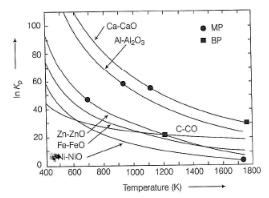Solution:

In the extraction of iron, at low temperature CO is effective and at high temperature carbon is more effective.

QUESTION: 15

Comprehension Type

Direction : This section contains a paragraph, describing theory, experiments, data, etc. Two questions related to the paragraph have been given. Each question has only one correct answer among the four given options (a), (b), (c) and (d).

Passage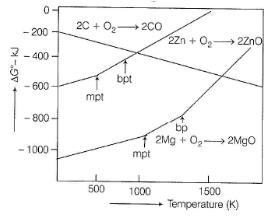Q.

To make the reduction ZnO+ C → Zn + CO spontaneous, temperature should be

Solution:

The temperature should be above the intersecting temperature.

QUESTION: 16

PassageQ.

At 1100°C, which reaction is spontaneous to a maximum extent ?

Solution:

At 1100° C the ΔG° for
ZnO + Mg → Zn + MgO, is more negative then other three reactions.

QUESTION: 17

Matching List Type

Direction : Choices for the correct combination of elements from Column I and Column II are given as options (a), (b), (c) and (d), out of which one is correct.

Q.

Match the Column I with Column II and mark the correct option from the codes given below.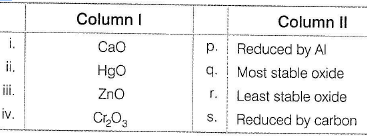Solution:

(i) → (q), (ii) → (r), (iii) → (s), (iv) → (p)

QUESTION: 18

Match the Column I with Column II and mark the correct option from the codes given below.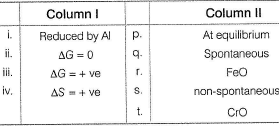Solution:

(i) → (r,t), (ii) → (p), (iii) → (s), (iv) → (q)

*Answer can only contain numeric values
QUESTION: 19

One Integer Value Correct Type

Direction : This section contains 5 questions. When worked out will result in an integer from 0 to 9 (both inclusive).

Q.

In the Gibbs equation at equilibrium the value of ΔG is

Solution:

ΔG = ΔH-T&S
At eqbm. (ΔG = 0)
0 = ΔH - 7ΔS ⇒ ΔH = TΔS

*Answer can only contain numeric values
QUESTION: 20

The reaction 3CaO + 2AI → Al2O3 + 3Ca is non-spontaneous. The number of electrons involved are

Solution:
*Answer can only contain numeric values
QUESTION: 21

The given reaction 2AI + Cr2O3 → Al2O3 + 2Cr is spontaneous. The number of electrons involved are

Solution:

6 electrons from 2 Al-atoms participate.

*Answer can only contain numeric values
QUESTION: 22

Among PbO, ZnO, CaO, Al2O3, SnO2, Cr2O3, WO3, HgO the oxides reduced by carbon are

Solution:

PbO, ZnO and SnO2 are reduced by carbon.

*Answer can only contain numeric values
QUESTION: 23

Cu2+ + Fe → Cu + Fe2+ for this reaction to calculate ΔG° the formula ΔG° = - nFE° is used. Here, value of n is

Solution:

ΔG°= -nFE°, n = number of electrons involved

QUESTION: 24

Statement Type

Direction : This section is based on Statement I and Statement II. Select the correct answer from the codes given below.

Q.

Statement I : Carbon is good reducing agent for sulphides.

Statement II : Carbon is poor reducing agent for iron oxide.

Solution:

Carbon is a good reducing agent for oxides, it is a poor reducing agent for sulphides. The reason why carbon reduces so many oxides at elevated temperature is that the ΔG°/T line for CO has a negative slope. There is no compound as analogous to CO with a steep negative ΔG°/T line. Thus sulphides are normally roasted in air to form oxides before reducing with carbon
2 MS + 3O→ 2MO + 2SO2

QUESTION: 25

Statement I : Carbon can reduce Fe2O3 to Fe at a temperature below 983 K.

Statement II : Iron has higher affinity toward oxygen than carbon.

Solution:

Below 983 K iron having higher affinity towards oxygen than carbon, carbon cannot reduce Fe2O3 to Fe.